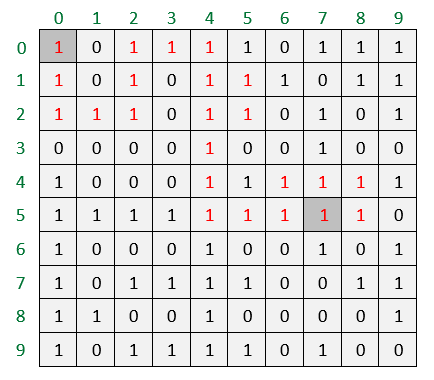# Find Longest Possible Route in a Matrix

Given a rectangular path in the form of binary matrix, find the length of longest possible route from source to destination position of the matrix by moving to only non-zero adjacent positions i.e. route can be formed from positions having their value as 1. Note there should not be any cycles in the output path.

For example, longest path from given source cell (0, 0) to destination cell (5, 7) for below matrix is of length 22.

(0, 0) -> (1, 0) -> (2, 0) -> (2, 1) -> (2, 2) -> (1, 2) -> (0, 2) ->
(0, 3) -> (0, 4) -> (1, 4) -> (1, 5) -> (2, 5) -> (2, 4) -> (3, 4) ->
(4, 4) -> (5, 4) -> (5, 5) -> (5, 6) -> (4, 6) -> (4, 7) -> (4, 8) ->
(5, 8) -> (5, 7)We can use Backtracking to solve this problem. We start from given source cell in the matrix and explore all four paths possible and recursively check if they will leads to the destination or not. We have to keep track of current distance of a cell from the source. We update the value of longest path found so far whenever destination cell is reached. If a path doesn’t reach destination or we have explored all possible routes from the current cell, we backtrack. To make sure that the path is simple and doesn’t contain any cycles, we keep track of cells involved in current path in an matrix and before exploring any cell, we ignore the cell if it is already covered in current path.

## C++

Output:

Maximum length path is 22

## Java

Output:

Maximum length path is 22(1 votes, average: 5.00 out of 5)Loading...

Please use our online compiler to post code in comments. To contribute, get in touch with us.
Like us? Please spread the word and help us grow. Happy coding 🙂Subscribe
Notify ofGuest
Suryansh

Is it possible to do better than this…Guest

What is the runtime for this? Since you have to find every path it must be exponential right? What would be the worst case runtime for this?Guest

Yes, the time complexity is exponential.